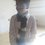# Another way of looking at A.P. (Derivatives)

An Arithmetic Progression is defined as a sequence such that the difference between two consecutive terms is constant. Hence the $$n^{\text{th}}$$ term of such a sequence is given by $$a_n=a+(n-1)d$$, where $$a$$ and $$d$$ denote the first term and the common difference respectively. So I was looking at an A.P. with this common difference as the rate of change of a term of an A.P..

If the $n^{\text{th}}$ term of an A.P. is given by $a_n=an+b$, then we have

$a_1=a+b~\text{and}~a_2=2a+b \\ \Rightarrow k=a_2-a_1=a \\ \boxed{k=a}$

where $k$ denotes the common difference. This is in sync with

$k=\dfrac{da_n}{dn}=\dfrac{d}{dn}(an+b)=a \\ \boxed{k=a}$

But it doesn't go hand in hand here if we look at the sums of $1,2,3,\dots$ terms of an A.P. from the beginning changing at the rate of the general term $a_n$. If $S_n=an^2+bn$ denotes the sum of first $n$ terms of an A.P. (it will be of this form as an existent formula for the sum of first $n$ terms of an A.P. which can be rearranged into this form is $\dfrac{n}{2}(2a+(n-1)d)$, where $a$ and $d$ are the first term and the common difference of an A.P. respectively), then we have

$a_1=S_1=a+b~\text{and}~a_1+a_2=S_2=4a+2b \\ \Rightarrow a_2 = S_2-S_1=3a+b \\ \Rightarrow k=a_2-a_1=2a \\ \Rightarrow a_n=(a+b)+(n-1)(2a)=b-a+2an \\ \boxed{a_n=b-a+2an}$

And if we go with derivatives, we have

$a_n=\dfrac{dS_n}{dn}=\dfrac{d}{dn}(an^2+bn)=2an+b \\ \boxed{a_n=2an+b}$

Can someone explain to me the disappearance of the $-a$? One interesting observation is that this does hold true.

$k=\dfrac{da_n}{dn}=\dfrac{d}{dn}(a_n)=\dfrac{d}{dn}\left(\dfrac{dS_n}{dn}\right)=\dfrac{d^2S_n}{dn^2} \\ k = \dfrac{d^2}{dn^2}(an^2+bn)=2a \\ \boxed{k=2a}$Note by Omkar Kulkarni
5 years, 10 months ago

This discussion board is a place to discuss our Daily Challenges and the math and science related to those challenges. Explanations are more than just a solution — they should explain the steps and thinking strategies that you used to obtain the solution. Comments should further the discussion of math and science.

When posting on Brilliant:

• Use the emojis to react to an explanation, whether you're congratulating a job well done , or just really confused .
• Ask specific questions about the challenge or the steps in somebody's explanation. Well-posed questions can add a lot to the discussion, but posting "I don't understand!" doesn't help anyone.
• Try to contribute something new to the discussion, whether it is an extension, generalization or other idea related to the challenge.

MarkdownAppears as
*italics* or _italics_ italics
**bold** or __bold__ bold
- bulleted- list
• bulleted
• list
1. numbered2. list
1. numbered
2. list
Note: you must add a full line of space before and after lists for them to show up correctly
paragraph 1paragraph 2

paragraph 1

paragraph 2

[example link](https://brilliant.org)example link
> This is a quote
This is a quote
    # I indented these lines
# 4 spaces, and now they show
# up as a code block.

print "hello world"
# I indented these lines
# 4 spaces, and now they show
# up as a code block.

print "hello world"
MathAppears as
Remember to wrap math in $$ ... $$ or $ ... $ to ensure proper formatting.
2 \times 3 $2 \times 3$
2^{34} $2^{34}$
a_{i-1} $a_{i-1}$
\frac{2}{3} $\frac{2}{3}$
\sqrt{2} $\sqrt{2}$
\sum_{i=1}^3 $\sum_{i=1}^3$
\sin \theta $\sin \theta$
\boxed{123} $\boxed{123}$

Sort by:

You can't differentiate it that way. It's the same reason why you can't differntiate $x^2 = x + x + x +\ldots + x$ to get $2x = 1 + 1 + 1 + \ldots + 1$.

- 5 years, 10 months ago

Sorry, lot of questions. I'm very much new to differentiation. 1. Why can't we differentiate in your example? 2. Is the fact that the other two things hold true a coincidence?

- 5 years, 10 months ago

What I have is a discrete case. The equation is only true for discrete number of $x$. You need to make sure it's continuous first before differentiating. No, it's not a coincidence, it's just the way it was set up to mislead people.

- 5 years, 10 months ago